# Intermediate Geometry : How to find an angle in a parallelogram

## Example Questions

2 Next →

### Example Question #11 : How To Find An Angle In A Parallelogram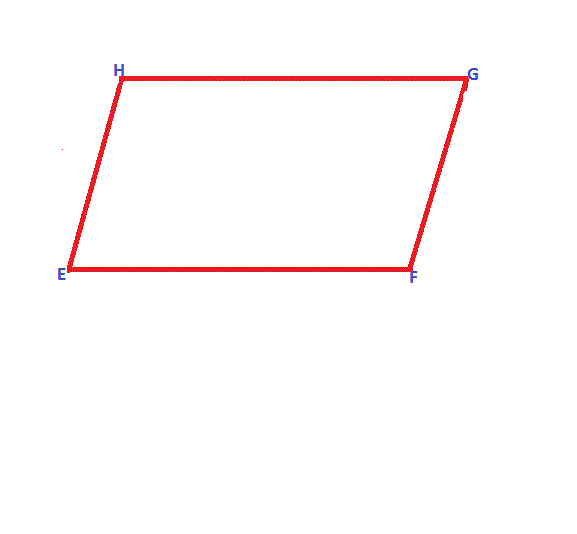In the parallelogram shown above, angle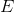isdegrees. Find the measure of angle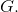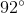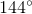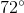Explanation:

A parallelogram must have equivalent opposite interior angles. Additionally, the sum of all four interior angles must equal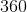degrees. And, the adjacent interior angles must be supplementary angles (sum ofdegrees).

Since, anglesand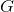are opposite interior angles, thus they must be equivalent.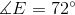, therefore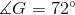### Example Question #12 : How To Find An Angle In A ParallelogramIn the parallelogram shown above, angleisdegrees. Find the sum of anglesand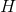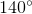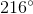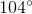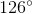Explanation:

A parallelogram must have equivalent opposite interior angles. Additionally, the sum of all four interior angles must equaldegrees. And, the adjacent interior angles must be supplementary angles (sum ofdegrees).

Thus, the solution is: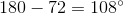Since both anglesandequal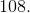There sum must equal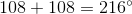### Example Question #13 : How To Find An Angle In A ParallelogramUsing the parallelogram above, find the sum of anglesand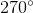Not enough information is provided to find an answer.Explanation:

A parallelogram must have equivalent opposite interior angles. Additionally, the sum of all four interior angles must equaldegrees.

Also, the adjacent interior angles must be supplementary angles (sum ofdegrees).

Since, anglesandare adjacent to each other they must be supplementary angles.

Thus, the sum of these two angles must equaldegrees.

### Example Question #14 : How To Find An Angle In A Parallelogram

A paralellogram as two angles that are 65 degrees and 115 degrees respectively.  What are the other two angles in the paralellogram?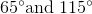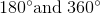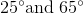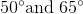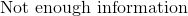Explanation:

This question is very simple to answer if you remember that ALL paralellograms have two pairs of equal and opposite angles, and that the four angles in any quadrilateral MUST add up to 360 degrees.

Because the angles given are different, we know that they are supplementary and the other two missing angles MUST be the same.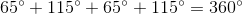### Example Question #15 : How To Find An Angle In A Parallelogram

Given: Regular Pentagon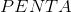with center. Construct segments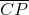and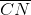to form Quadrilateral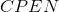.

True or false: Quadrilateralis a parallelogram.

True

False

False

Explanation:

Below is regular Pentagonwith center, a segment drawn fromto each vertex - that is, each of its radii drawn.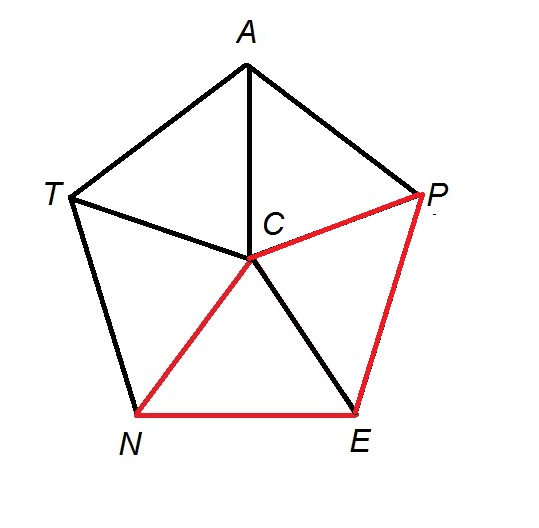The measure of each angle of a regular pentagon can be calculated by settingequal to 5 in the formula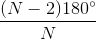and evaluating: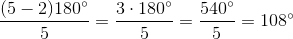Specifically,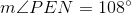By symmetry, each radius bisects one of these angles. Specifically,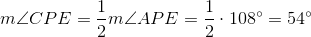By the Same-Side Interior Angles Theorem, consecutive angles of a parallelogram are supplementary - that is, their measures total. However,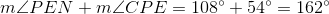,

violating these conditions. Therefore, Quadrilateralis not a parallelogram.

### Example Question #16 : How To Find An Angle In A Parallelogram

Given: Quadrilateralsuch that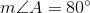and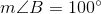.

True or false: It follows that Quadrilateralis a parallelogram.

False

True

False

Explanation: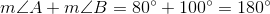, making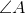and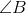supplementary. By the Converse of the Same Side Interior Angles Theorem, , it does follow that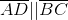. However, without knowing the measures of the other two angles, nothing further can be concluded about Quadrilateral. Below are a parallelogram and a trapezoid, both of which have these two angles of these measures.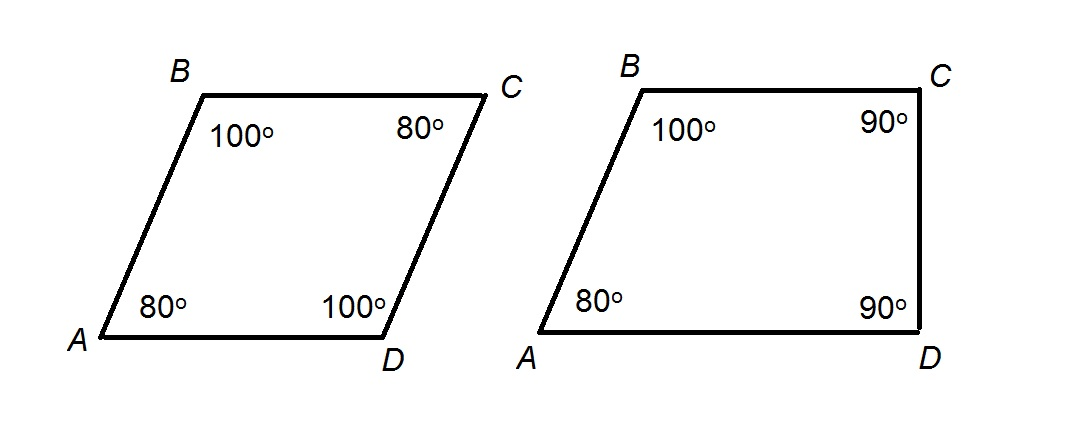### Example Question #17 : How To Find An Angle In A Parallelogram

Given: Parallelogramsuch that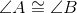and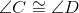.

True or false: It follows that Parallelogramis a rectangle.

True

False

True

Explanation:

By the Same-Side Interior Angles Theorem, consecutive angles of a parallelogram can be proved to be supplementary - that is, their angle measures total. Specifically,andare a pair of supplementary angles. Since they are also congruent, it follows that both are right angles. For the same reason,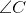and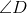are also right angles. The parallelogram, having four right angles, is a rectangle by definition.

### Example Question #18 : How To Find An Angle In A Parallelogram

Given: Rectanglewith diagonals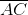and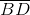intersecting at point.

True or false: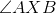must be a right angle.

True

False

False

Explanation:

The diagonals of a parallelogram are perpendicular - and, consequently,is a right angle. - if and only if the parallelogram is a rhombus, a figure with four sides of equal length. Not all rectangles have four congruent sides.  Therefore,need not be a right angle.

### Example Question #19 : How To Find An Angle In A Parallelogram

Given: Parallelogramsuch that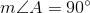.

True or false: Parallelogrammust be a rectangle.

False

True